Java Generics Tutorial With Example | The Basics of Java Generics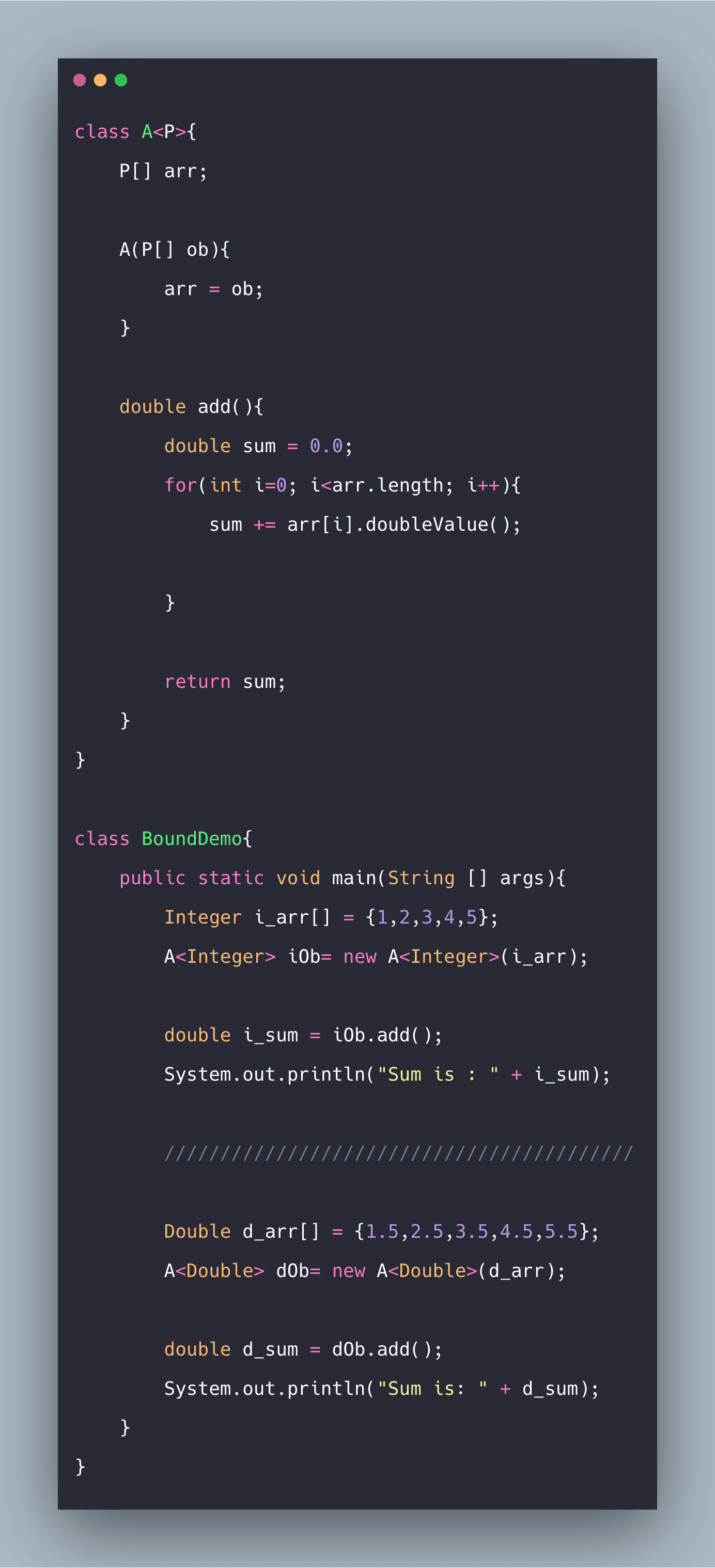Java Generics Tutorial With Example | The Basics of Java Generics is today’s topic. The Java Generics features were added to the Java language from Java 5. Generics add a way to specify particular types to general-purpose classes and methods that operated on Object before. Java generics provide compile-time type checking and removing the risk of ClassCastException that was common while working with collection classes.

Java Generics Tutorial

Generics were introduced to Java by JDK 5. It changed Java in two important ways – it added a syntactical element to the language, and, it caused changes to many of the classes and methods in the core API. Generics makes it possible to create classes, interfaces, and methods that can work with various kinds of data in a type-safe manner. It is often found that the same algorithm needs to be implemented for different types of data. So rather than creating different classes or methods for different data types, it is convenient to create classes or methods which are generic.

A generic class in Java is the class that operates on a parameterized type. The following example demonstrates the use of parameterized type aptly.

``````// Example.java

class GenericClass<P>{
P ob;

GenericClass(P o){
ob=o;
}

P getobj(){
return ob;
}

void getType(){
System.out.println("Type of Parameter is " + ob.getClass().getName());
}
}

class Example{
public static void main(String [] args){
GenericClass<Double> dOb;

dOb = new GenericClass<Double>(100.23);
dOb.getType();

double d= dOb.getobj();
System.out.println("Value: " + d);
System.out.println();

////////////////////////////////////

GenericClass<String> strOb = new GenericClass<String>("Generics Demo");

strOb.getType();

String str = strOb.getobj();
System.out.println("Value: "+ str);
}
}``````

See the following output.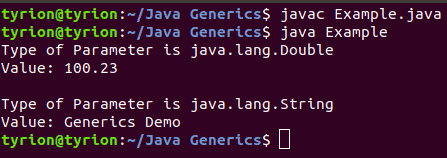Here, we create a generic class GenericClass with type parameter P. This acts as a placeholder for the actual type parameter that will be passed to GenericClass when an object is created. The name of the type parameter is contained within angle brackets <>.  Then P is used to declare an object ob.

Therefore, ob will be an object of the type passed to P.  In the example above, when the first instance of GenericClass is created, type Double is passed to P and therefore, ob is of type Double. For the second instance, however, type String is passed to P, making ob of type String here.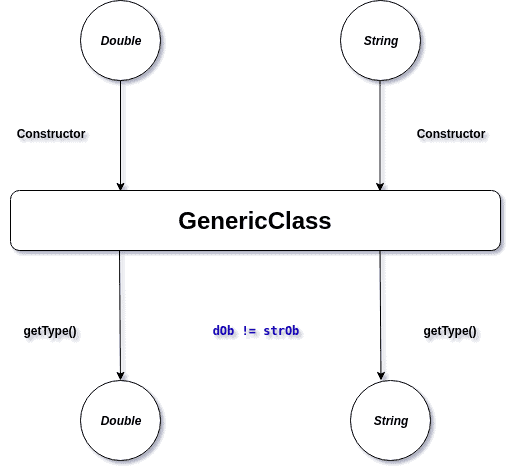Although the use of generics is very convenient, one must keep a few things in mind while working with generics.

Generics work only with reference types: When an instance of a generic type is declared, the type parameter used must be of a reference type. A primitive type cannot be used, therefore, while instantiating a generic type.

For example, the following declaration will be illegal:

``GenericClass<char> charOb = new GenericClass<char>(‘a’);``

Generic types differ based on their type of arguments: In the previous example, the different versions of GenericClass are instantiated, one with reference dOb and the other with reference strOb. Although both dOb and strOb are of the type GenericClass<P>,  the statement dOb = strOb will produce a compilation error.

#Java Generic Type

Java Generic Type Naming convention helps us to understand code quickly, and having the naming convention is one of the best practices of Java programming language. So generics also comes with its naming conventions. Usually, the type parameter names are single, uppercase letters to make it easily distinguishable from the java variables. Commonly used type parameter names are.

• E – Element (used extensively by the Java Collections Framework, for example, ArrayList, Set, etc.)
• K – Key (Used in Map)
• N – Number
• T – Type
• V – Value (Used in Map)
• S, U, V, etc. – 2nd, 3rd, 4th types

#Java Generics Wildcards

Question mark (?) is the wildcard in tbe generics and represent an unknown type. The wildcard can be used as the type of the parameter, field, or local variable and sometimes as the return type. We can’t use the wildcards while invoking a generic method or instantiating a generic class.

The question mark in Java represents wildcards “?” and they are used to refer to an unknown type. The Wildcards are particularly useful when using the generics and can be used as the parameter type, but first, there is an important note to consider.

It is known that Object is the supertype of all the Java classes; however, a collection of Object is not the supertype of any collection.

For example, the List<Object> is not the supertype of List<String> and assigning a variable of the type List<Object> to the variable of type List<String> will cause a compiler error. This is to prevent the possible conflicts that can happen if we add different types to the same collection.

The Same rule applies to any collection of a type and its subtypes. Consider the following example.

``````public static void paintAllBuildings(List<Building> buildings) {
buildings.forEach(Building::paint);
}``````

If we imagine the subtype of Building, for example, the House, we can’t use this method with a list of House, even though House is the subtype of Building. If we need to use the above method with type Building and all its subtypes, then the bounded wildcard can do the magic.

``````public static void paintAllBuildings(List<? extends Building> buildings) {
...
}
``````

#Java Generics Type Erasure

Generics in the Java was added to provide type-checking at compile time, and it has no use at the run time, so java compiler uses the type erasure feature to remove all the generics type checking code in the byte code and insert the type-casting if necessary. Type erasure ensures that no new classes are created for parameterized types; consequently, generics incur no runtime overhead.

For example, if we have the generic class like below.

``````public class Test<T extends Comparable<T>> {

private T data;
private Test<T> next;

public Test(T d, Test<T> n) {
this.data = d;
this.next = n;
}

public T getData() { return this.data; }
}``````

The Java compiler replaces the bounded type parameter T with the first bound interface, Comparable, as below code.

``````public class Test {

private Comparable data;
private Test next;

public Node(Comparable d, Test n) {
this.data = d;
this.next = n;
}

public Comparable getData() { return data; }
}``````

#Creating a Generic Class with Two Type Parameters

A generic class is not limited to use only one type of parameter. More than one type parameter can be used in a generic class by using a comma-separated list. The following code example demonstrates how to use two type parameters in a generic class.

See the following code example.

``````// ExampleTwo.java

class TwoGenericClass<P, Q>{
P ob1;
Q ob2;

TwoGenericClass(P o1, Q o2){
ob1=o1;
ob2=o2;
}

void getType(){
System.out.println("Type of P is: " + ob1.getClass().getName());
System.out.println("Type of Q is: " + ob2.getClass().getName());

}

P getobj1(){
return ob1;
}

Q getobj2(){
return ob2;
}

}

class ExampleTwo{
public static void main(String [] args){
TwoGenericClass<Double, String> ob = new TwoGenericClass<Double, String>(100.22,"Generics Demo");

ob.getType();

double d = ob.getobj1();
System.out.println("Value: " + d);

String s = ob.getobj2();
System.out.println("Value: " + s);
}
}
``````

See the following output.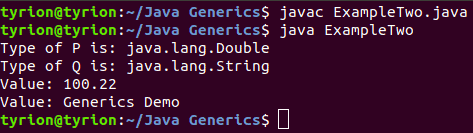Here, a second type parameter Q accommodates the use of more than one type parameter in GenericClass. Note how both the type parameters are to be declared (TwoGenericClass<Double, String>) while instantiating the generic class.

#The use of Bounded Types

In the above examples, the placeholders used in the generic classes can be replaced by any parameter type. Although this can be helpful in many situations, in some situations, we can need some restrictions on the type of type parameters that can be allowed for a generic class in question.

``````// BoundDemo.java

class A<P>{
P[] arr;

A(P[] ob){
arr = ob;
}

double sum = 0.0;
for(int i=0; i<arr.length; i++){
sum += arr[i].doubleValue();

}

return sum;
}
}

class BoundDemo{
public static void main(String [] args){
Integer i_arr[] = {1,2,3,4,5};
A<Integer> iOb= new A<Integer>(i_arr);

System.out.println("Sum is : " + i_sum);

//////////////////////////////////////////

Double d_arr[] = {1.5,2.5,3.5,4.5,5.5};
A<Double> dOb= new A<Double>(d_arr);

System.out.println("Sum is: " + d_sum);
}
}``````

In the above example, the method double add() is defined to add the elements of the array using the method doubleValue() which is a method declared by Number. Therefore, it supports only objects of Number or its subclass.

Since the code written above is “generic,” and any type parameter is allowed to take the place of P, the Java compiler doesn’t allow the code to compile.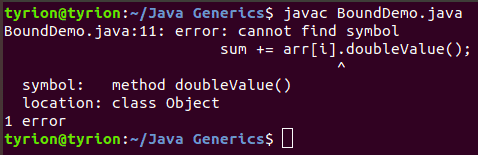To fix this issue, one has to specify that we are expecting only type parameter of class Number or that of any of its subclass to be used in Class A.

This can be done using the extends keyword:

``class A <P extends Number>``

See the following code example.

``````// Bound2Demo.java

class A<P extends Number>{
P[] arr;

A(P[] ob){
arr = ob;
}

double sum = 0.0;
for(int i=0; i<arr.length; i++){
sum += arr[i].doubleValue();

}

return sum;
}
}

class Bound2Demo{
public static void main(String [] args){
Integer i_arr[] = {1,2,3,4,5};
A<Integer> iOb= new A<Integer>(i_arr);

System.out.println("Sum is : " + i_sum);

//////////////////////////////////////////

Double d_arr[] = {1.5,2.5,3.5,4.5,5.5};
A<Double> dOb= new A<Double>(d_arr);

System.out.println("Sum is: " + d_sum);
}
}``````

See the below output.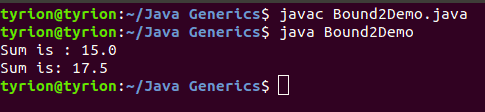Now since Number bounds the type P, the Java compiler knows that all objects of type P can call the method doubleValue() as it is a method declared by Number.

Multiple Bounds

A type can also have multiple upper bounds as follows.

``<T extends Number & Comparable>``

If one of the types that are extended by T is a class (i.e., Number), it must be put first in the list of bounds. Otherwise, it will cause a compile-time error.

#Java Generic Interface

Comparable interface is an excellent example of Generics in interfaces, and it’s written as the following.

``````package java.lang;
import java.util.*;

public interface Comparable<T> {
public int compareTo(T o);
}``````

Similarly, we can create generic interfaces in java. We can also have multiple type parameters as in Map interface. Again we can provide parameterized value to a parameterized type also, for example, new HashMap<String, List<String>>(); is valid. You can read Java HashMap.

Generics in Java, therefore, allows the programmer to make use of the same functionality across a range of data types without having to duplicate code – saving both the time needed to code, as well as, making the code more readable; and at the same time do so by ascertaining certain restrictions/bounds wherever required.

Finally, Java Generics Tutorial With Example | The Basics of Java Generics is over.